﻿ (k,k-1)-双正则可图序列的公平划分

# (k,k-1)-双正则可图序列的公平划分Judicious Balanced Bipartitions of (k,k-1)-Biregular Graphic Degree Sequence

Abstract: Let π= （d1,d2,…,dn be a graphic sequence of nonnegative integers and π12 are two sequences that are obtained by partitioning the elements of π into two sets. A balanced bipartition of π is a bipartition π12 such that -1≤|π1|-| π2|≤1, where |πi|(i=1,2) is denoted to the number of elements of πi. In this paper, let k and m be positive integers, we determine the values Ψmax(π) and Ψmin(π) of (k,k-1)-biregular graphic sequence π= (km,(k-1)m).

1. 引言

${V}_{1},{V}_{2}$ 是图G的一个二部划分，如果 $-1\le |{V}_{1}|-|{V}_{2}|\le 1$ ，则称 ${V}_{1},{V}_{2}$ 是G的一个二部平衡划分。对于 $i=1,2$$e\left({V}_{i}\right)$ 表示两个端点都在 ${V}_{i}$ 中的边的数目， $e\left({V}_{1},{V}_{2}\right)$ 表示两个端点分别在顶点子集 ${V}_{1},{V}_{2}$ 中的边数。通常 $e\left({V}_{1},{V}_{2}\right)$ 用来表示平衡二部划分的大小。图G的一个最大(最小)平衡二部划分 ${V}_{1},{V}_{2}$ 是图G的所有平衡二部划分中 $e\left({V}_{1},{V}_{2}\right)$ 的值达到最大(最小)。与最大，最小平衡划分问题不同，公平划分问题是寻找图G的一个划分，使得多个分量同时进行优化。

2. 主要定理及引理

$\underset{i=1}{\overset{t}{\sum }}{d}_{i}\le t\left(t-1\right)+\underset{j=t+1}{\overset{n}{\sum }}\mathrm{min}\left\{{d}_{i},t\right\},\text{\hspace{0.17em}}1\le t\le n$

$P:=\left({p}_{1},{p}_{2},\cdots ,{p}_{m}\right)$$Q:=\left({q}_{1},{q}_{2},\cdots ,{q}_{n}\right)$ 是两个非负整数序列。如果存在一个简单二部图 $G\left[X,Y\right]$ 使得X和Y中的顶点度分别是 $\left({p}_{1},{p}_{2},\cdots ,{p}_{m}\right)$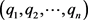，那么称序列对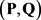是二部可图的，并称二部图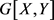的一个实现。Gale  和Ryser  分别独立地给出了关于二部可图序列的刻划定理。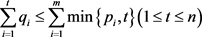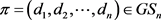，若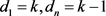，则称π是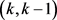-双正则可图的。本文主要给出双正则可图序列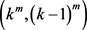的公平划分的上下界。

3. (k, k − 1)-双正则可图序列的公平划分的上界

1) 若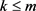，则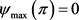2) 若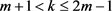，则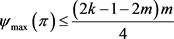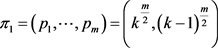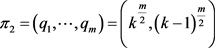，那么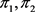是π的一个平衡二部(1)(2)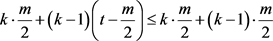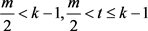，由(1)和(2)得，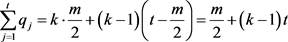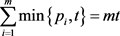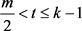可得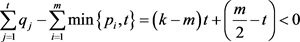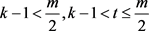，由(1)和(2)得，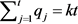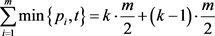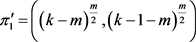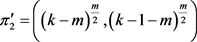。由于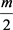是偶数，所以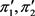的度和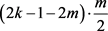为偶数。由引理2.3知，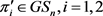。设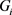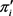的一个实现且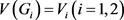。令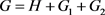。容易验证G是π的一个实现，且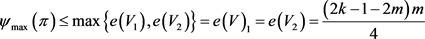4. (k, k-1)-双正则可图序列的公平划分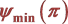的下界

1) 若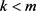，则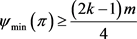2) 若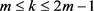，则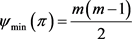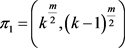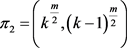，则是π的一个平衡二部划分。由于是偶数，所以的度和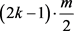为偶数。又由引理2.3可得，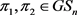。设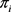的一个实现且，令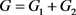。容易验证G是π的一个实现，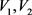是G的一个平衡二部划分且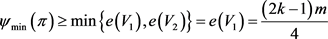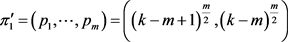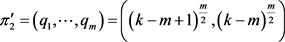。这里，(3)(4)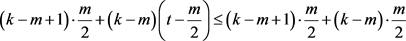，由(3)和(4)得，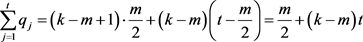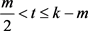可知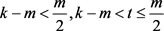，由(3)和(4)得，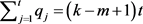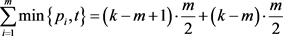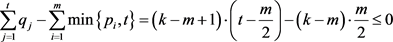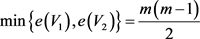Bondy, J.A. and Murty, U.S.R. (1976) Graphy Theory with Applications. Macmillan Ltd Press, New York.
https://doi.org/10.1007/978-1-349-03521-2

 Erdös, P. and Gallai, T. (1960) Graphs with Prescribed Degrees of Vertices. Matematikai Lapok, 11, 264-274.

 Gale, D. (1957) A Theorem on Flows in Networks. Pacific Journal of Mathematics, 7, 1073-1082.
https://doi.org/10.2140/pjm.1957.7.1073

 Ryser, H.J. (1957) Combinatorial Properties of Matrices of Zeros and Ones. Canadian Journal of Mathematics, 9, 371-377.
https://doi.org/10.4153/CJM-1957-044-3

 Yin, J.H. and Li, J.S. (2005) Two Sufficient Conditions for a Graphic Sequence to Have a Realization with Prescribed Clique Size. Discrete Mathematics, 301, 218-227.
https://doi.org/10.1016/j.disc.2005.03.028

Top